Question Pool Reactivity series & Electrochemistry

# Reactivity series & Electrochemistry - SAMAGRA Question Pool & Answers | Class 10 English Medium

Kerala Syllabus SAMAGRA SCERT SAMAGRA Question Pool for Class 10 English Medium Chemistry Reactivity series & ElectrochemistryQn 1.

Experiments related to displacement reaction are given below

1) A Silver rod is dipped in CuSO4 .

2) A Zinc rod is dipped in CuSO4

In which case does displacement reaction occur .why?

Experiment- 2

Reactivity of Zn is greater than

Get Free Study Materials + 1 Week Free Trial of BrainsPrep Class 10 English Medium Tuition

Qn 2.

On electrolysis of fused sodiumchloride sodium is formed at the cathode and chlorine at the anode

a) Write the equation of reactions occuring at the anode and cathode

b) If aqueous solution of sodium chloride is electrolysed,what will be the product formed at the cathode?

a) Cathode -‍ Na+  1e- → Na

Anode 2Cl- → Cl2 +

Get Free Study Materials + 1 Week Free Trial of BrainsPrep Class 10 English Medium Tuition

Qn 3.

Diagram of Galvanic cell is given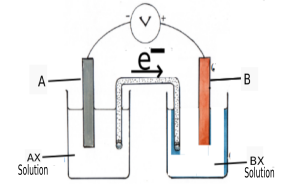a) Which is the anode of thts cell ?

b) Write the equation of the cathode reaction

(Valency of metals : 2)

a) A

b) B2+ + 2e- →

Get Free Study Materials + 1 Week Free Trial of BrainsPrep Class 10 English Medium Tuition

Qn 4.

Analyse the given equation and answer the questions.

Zn+ Cu2+SO42- →Zn2+SO42- + CuO

a) Which metal is oxidised?

b) Is the above reaction a redox one. Why?

a) Zn

b) yes,

In this reaction Zn is oxidised and

Get Free Study Materials + 1 Week Free Trial of BrainsPrep Class 10 English Medium Tuition

Qn 5.

Some observations of the reaction of metals Al, Na ,Fe, Cu with water are given

1. One metal reacted with cold water to form an alkali and a gas

2. Another metal reacted only with steam to form a gas.

a) Which is the gas formed when metals react with water?

b) Which of the above metals give  the observations 1 and 2?

c) Write the equation of the reaction which gave the first observation.

a) H2

b) Observation (1) Na

Observation (2) Fe

c) 2Na + 2H2O →2NaOH +

Get Free Study Materials + 1 Week Free Trial of BrainsPrep Class 10 English Medium Tuition

Qn 6.

The decreasing order of reactivity of some metals are given

Mg>Al>Zn>Fe>Cu>Au

a) Name any one metal that cannot displace hydrogen from dil. HCl?

b) Which metal reacts only with steam to displace hydrogen from water?

c) Which metal can displace all other metals from their salt solutions?

a) Cu (or) Au

b) Fe

c) Mg

Get Free Study Materials + 1 Week Free Trial of BrainsPrep Class 10 English Medium Tuition

Qn 7.

A newly cut surface of sodium and a rubbed surface of zinc appears shiny

a) Which of the above loses its lustre easily?

b) Write the equation of any one reaction which causes loosing of its lustre

c)  Compare  the the reactivity of the two metals?

a) Na

b) 4Na + O2 →2Na2O (or)

2Na + 2H2O →2NaOH +

Get Free Study Materials + 1 Week Free Trial of BrainsPrep Class 10 English Medium Tuition

Qn 8.
 cell Positive electrode Negative electrode Galvanic cell Cathode (a) ............................... Electrolytic cell (b) ................................ (c) ................................

a) anode

b) anode

c)

Get Free Study Materials + 1 Week Free Trial of BrainsPrep Class 10 English Medium Tuition

Qn 9.

Zn + CuSO4 → ZnSO4 + Cu

a)Write down the equation of oxidation reaction

b) Is it a redox reaction? why?

a) Zn+ →Zn2+ + 2e(Oxidation)

b) Yes.Because zinc undergoes oxidation and copper undergoes

Get Free Study Materials + 1 Week Free Trial of BrainsPrep Class 10 English Medium Tuition

Qn 10.

A Zinc rod is dipped in CuSO4 solution. What happens to the colour of the solution? Explain with the help of equation showing the reaction taking place in the test tube

Zn + CuSO4 → ZnSO4 + Cu

The blue colour of CuSOsolution is due to the presence

Get Free Study Materials + 1 Week Free Trial of BrainsPrep Class 10 English Medium Tuition

Qn 11.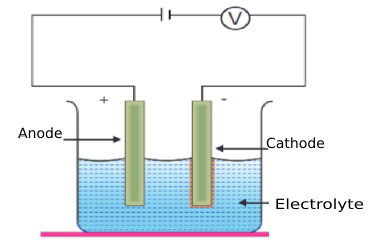A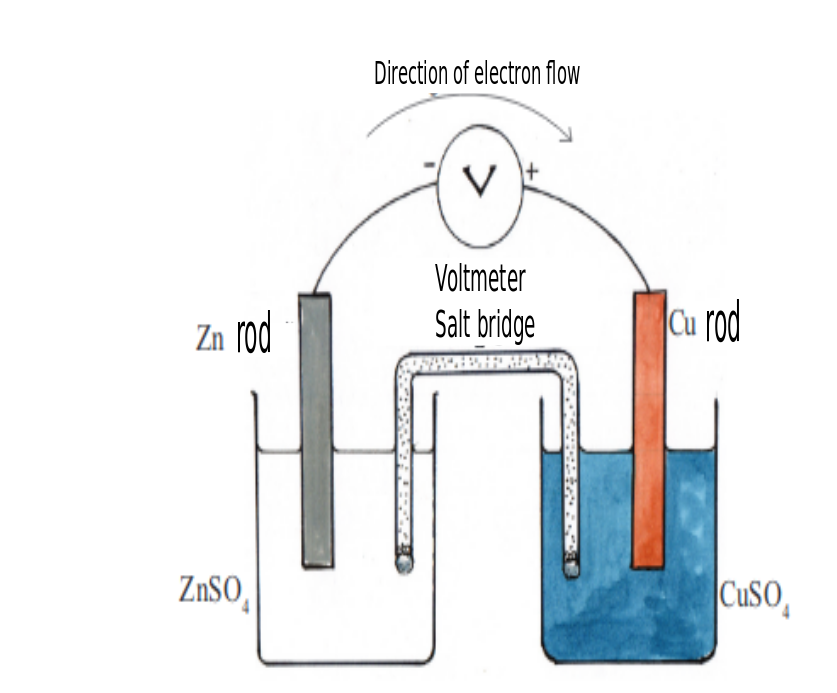B

a)Two type of cells are represented in figure A and B.What are they?

b) What is the energy change taking place in the cell B ?

c) Which is the positive electrode in B ??

a) Fig A -Electrolytic cell

Fig B -‍ Galvanic cell

b) Chemical energy is converted to Electrical energy

c) Cu

Get Free Study Materials + 1 Week Free Trial of BrainsPrep Class 10 English Medium Tuition

Qn 12.

NaCl crystals, sugar, moltenNaCl ,aqueous solution of NaCl are given.

Which of the above conduct electricity ?why?

Molten NaCl and solution of NaCl

They conduct electricity because they contains ions which are free to

Get Free Study Materials + 1 Week Free Trial of BrainsPrep Class 10 English Medium Tuition

Qn 13.

Electricity is passed through molten sodiumchloride and sodiumchloride solution

a)  Compare the reactions taking place at each electrodes and complete the table

 Electrolyte Positive electrode Negative electrode Molten sodiumchloride Cl2 (a) ..................... sodiumchloride solution (b) ................................ H2

b) Write equation of the reaction taking place at the positive electrode if molten KCl is used instead of molten NaCl?

a) (a) Na (b) Cl2

b) 2Cl- → Cl2 +

Get Free Study Materials + 1 Week Free Trial of BrainsPrep Class 10 English Medium Tuition

Qn 14.

Correct the given wrong statements, if any

a) In a Gavanic cell electrical energy is converted to chemical energy

b) The reactivity of cathode in a galvanic cell will be less than that of the anode

c) In a Gavanic cell electrons flow from cathode to anode

d) Oxidation take place at anode

a and c are wrong statements

a)In a Gavanic cell chemical energy is converted to electrical energy

c)In a Gavanic cell electrons flow from anode to

Get Free Study Materials + 1 Week Free Trial of BrainsPrep Class 10 English Medium Tuition

Qn 15.

Three metal pieces are dipped in water taken in three test tubes .Adrop of phenolphthalein is added to each test tube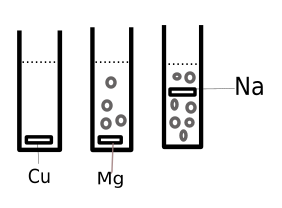a) Which metal forms pink colour on reaction with cold water?

b) Which metal gives pink colour only on heating ?

c) Write the balanced equation for the reaction taking place in any one test tube.

a) Na

b) Test tube B

c) 2Na + 2H2O →2NaOH + H2

OR Mg + 2H2O →Mg(OH)2 +

Get Free Study Materials + 1 Week Free Trial of BrainsPrep Class 10 English Medium Tuition

Qn 16.

Name the product formed at the cathode on electrolysis of molten KCl ?

K

Get Free Study Materials + 1 Week Free Trial of BrainsPrep Class 10 English Medium Tuition

Qn 17.
• The element A generally show +1 oxidation state If we electrolyse molten chloride of this element

a) What is the energy change taking place in an electrolytic cell?

• c) Name the product formed at the positive electrode?

• b) Write down the equation showing the oxidation reaction

a) Electrical energy is converted in to chemical energy

b) Cl2

b) Na+ 2e

Get Free Study Materials + 1 Week Free Trial of BrainsPrep Class 10 English Medium Tuition

Qn 18.

A piece of magnesium ribbon is dipped in CuSO4 solution. After some time Cu is found to get deposited on the ribbon

a) Write oxidation reaction taking place here?

b)Write the equation showing the redox reaction taking place in the test tube?

c)If a Ag rod is dipped instead of Mg ribbon,does any change in colour occur to the solution.why?

a) Mg → Mg2+ + 2e-

b) Mg + Cu2+→ Mg2++Cu

c) No colour change. Ag is less reactive than

Get Free Study Materials + 1 Week Free Trial of BrainsPrep Class 10 English Medium Tuition

Qn 19.

Figure of an electrolytic cell is given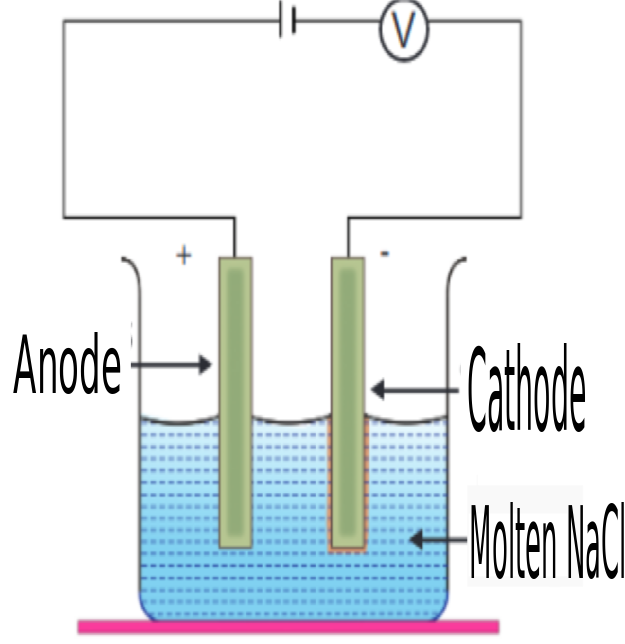a) Which is the product obtained at the cathode?

b) Write the equation of the chemical reaction taking place at anode

c) Write any two instances where electrolysis is made use of.

a) Na

b) 2Cl- → Cl2 + 2e-

c) Electroplating, Refining of

Get Free Study Materials + 1 Week Free Trial of BrainsPrep Class 10 English Medium Tuition

Qn 20.

Figure of an electrolytic cell is given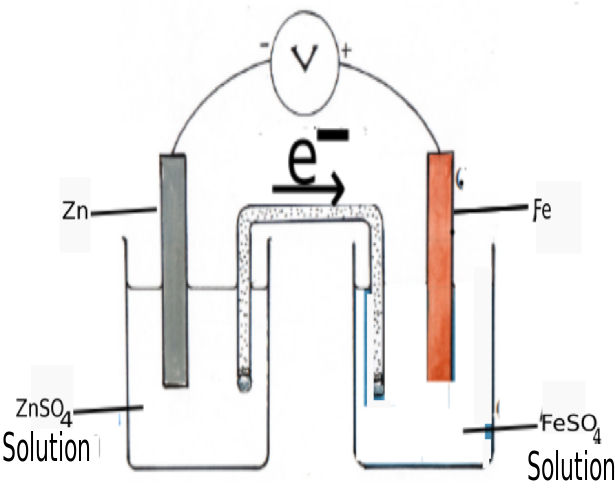a) Write the equation of the reduction reaction take place in the cell

b) If the flow of electrons in Mg-Zn cell is in opposite direction as that of Zn-Fe cell shown above, arrange the metals Zn,Mg,Fe in the ascending order of their reactivity

a) Fe2+ + 2e- → Fe

b)

Get Free Study Materials + 1 Week Free Trial of BrainsPrep Class 10 English Medium Tuition

Qn 21.

Equation of the reaction of iron rod and CuSOsolution is given below.

CuSO4 + Fe →FeSO4 + Cu

a) Write the eqation showing the reduction reaction taking place here.

b) Will displacement reaction take place by using ZnSO4 instead of CuSO4 ?Give reason

a) Cu2+ + 2e- → Cu

b) No , Zn is more reactive than

Get Free Study Materials + 1 Week Free Trial of BrainsPrep Class 10 English Medium Tuition

Qn 22.

Some metals are arranged in the decreasing order of their reactivity.

Mg>Zn>Pb>Cu>Ag

a) Which will be the anode of Zn- Cu Galvanic cell ?

b) Write the equation of the redox reaction taking place in the above cell?

a) Zn

b) Zn + Cu2+ → Zn2+ +

Get Free Study Materials + 1 Week Free Trial of BrainsPrep Class 10 English Medium Tuition

Qn 23.

Which is the product formed at cathode on electrolysis of molten NaCl?

Sodium (Na)

Get Free Study Materials + 1 Week Free Trial of BrainsPrep Class 10 English Medium Tuition

Qn 24.
• Analysis the following reactions and answer the following questions.

(Hint : Order of reactivity Mg>Zn>Fe>Cu)

Activity 1 : A copper rod is dipped in FeSO4 solution

Activity 2 : A Zinc rod is dipped in FeSO4 solution

a) In which test tube does displacement reaction take place ?

b) Write the redox reaction taking place here

a) Activity 2

b) Zn + Fe2+ → Zn2+ +

Get Free Study Materials + 1 Week Free Trial of BrainsPrep Class 10 English Medium Tuition

Qn 25.

The equation of the redox reaction taking place in a Galvanic cell is given below.

Cu + 2Ag+ →Cu2+ + 2Ag

a) Write the equation showing the reduction reaction

b)Draw the figure of the Galvanic cell

Get Free Study Materials + 1 Week Free Trial of BrainsPrep Class 10 English Medium Tuition

Qn 26.

Observe the figure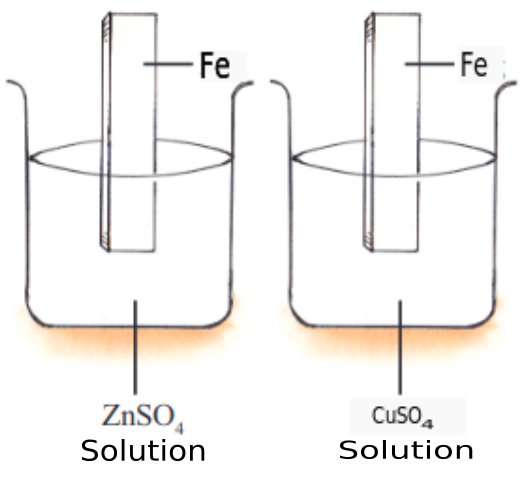(A )                          ( B)

(Hint:Order of reactivity Na>Mg>Zn>Fe>Cu)

a) The Fe rod dipped in which beaker shows a change in colour?

b) Write the equation for the oxidation reaction taking place

Get Free Study Materials + 1 Week Free Trial of BrainsPrep Class 10 English Medium Tuition

Qn 27.

Zn, Cu,Ag  rods and solutions of AgNO3 , CuSOZnSO4 and MgSO4 are given . How many Galvanic cells can be constructed from this? Complete the table.

(Mg>Zn>Fe>Cu)

 cell Anode Cathode Zn-Cu Zn Cu

Three types

Get Free Study Materials + 1 Week Free Trial of BrainsPrep Class 10 English Medium Tuition

Qn 28.

Some electrodes and salt solutions are shown.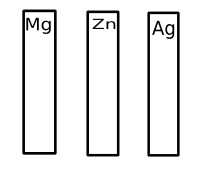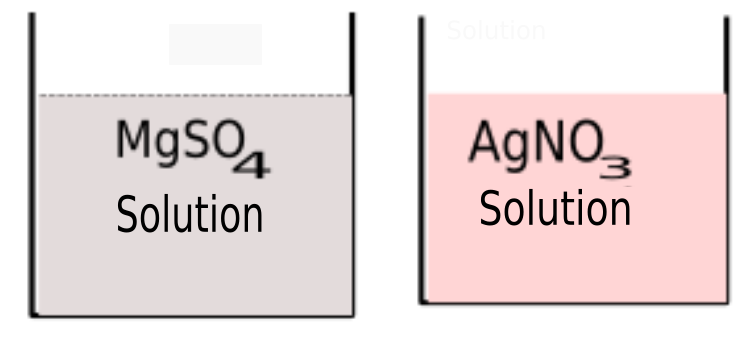a) Which is the Galvanic cell that can be constructed from the above?

b) What are the anode and cathode of the cell?

c) Write equaton of the reaction that takes place at anode ?

a) Mg – Ag cell

b) Anode Mg, Cathode Ag

c) Mg →Mg2+ +

Get Free Study Materials + 1 Week Free Trial of BrainsPrep Class 10 English Medium Tuition

Qn 29.

The diagram of a galvanic cell is given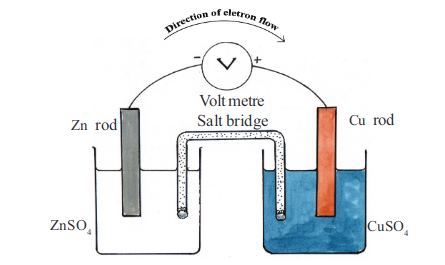a) What are the anode and cathode of the cell

b) Write the equation of the cathodic reaction

c) Write the eqation of the redox reaction taking place in the cell

a) Anode Zn , Cathode Cu

b) Cu2+ + 2e- → Cu

c) Zn + Cu2+ → Zn2+ +

Get Free Study Materials + 1 Week Free Trial of BrainsPrep Class 10 English Medium Tuition

Qn 30.

Reaction of some metals with water is given in the table( symbols are not real)

 Metal Reaction A Reaction with steam B Does not react C Reacts vigourously even with cold water D React with hot water

a)Based on the above reaction ,aarrange the given metals in the decreasing order of their reactivity

b)If a galvanic cell is constructed using A and B as electrodes, which is the anode???

c) Write the equation of the reaction taking place at electrode B of the cell

(valency of B=2)

a) C>D>A>B

b) A

c) B2+ + 2e-

Get Free Study Materials + 1 Week Free Trial of BrainsPrep Class 10 English Medium Tuition

Qn 31.

Sodium vigourously reacts with cold water

a) Which is the gas formed in the reaction?

b) Write the balanced chemical eqation of the reaction

c) If two drops of phenolphthalein are added to the test tube ,what can you observe?

a) Hydrogen

b) 2Na + 2H2O → 2NaOH + H2

c) Solution becomes

Get Free Study Materials + 1 Week Free Trial of BrainsPrep Class 10 English Medium Tuition

Qn 32.

Which among the given metals does not react with dilute acids ?

Copper

Get Free Study Materials + 1 Week Free Trial of BrainsPrep Class 10 English Medium Tuition

Qn 33.

Electricity is passed through sodium chloride solution taken in a beaker.

a) Which is the substance formed at the cathode?

b)Which substance gets discharged at the anode?

c) The reaction taking place at one electrode is
2H2O + 2e- → H2 + 2OH-
At which electrode this reaction takes place?

d) What will be the nature of the solution after electrolysis?

(Acidic / Neutral‍ / Alkaline‍)

a) H2

b) Cl-

c) At cathode‍

d)